by Brandon Rhodes • Home

# Animating Ptolemy’s Equant with Python, SVG, and CSS¶

You will recall my previous blog post that tried to build the necessary scaffolding for me to finally write up my 2017 PyCon Ireland keynote on the structure of the Medieval universe. It ran into several problems with matplotlib animations — but, having written that post, I realized that the problem ran deeper.

How could any animation show a Solar System, when a Solar System’s motion never exactly repeats? The orbital periods of the planets aren’t exact multiples of each other, and don’t provide a moment when the planets reach their original positions and the animation can start over again. At whatever moment an animation finished and looped back to the beginning, the planets would visibly and jarringly jump back to their original position.

But then I remembered that modern browsers support animation directly, and thought: could a python script produce an SVG diagram with a separate CSS animation for each planet, that repeated each time that specific planet finished a revolution?

The result would be an animated Solar System that fits into a few thousand bytes, would render with perfect clarity, and runs continuously for as long has the viewer was willing to watch!

But there’s a problem.

The CSS animation mechanism is perfect for the simplest possible planetary orbit: uniform circular motion. Here’s a simple SVG diagram in which a planet and the line connecting it to the origin are grouped within a single `<g>` element.

```%pylab inline
from IPython.display import HTML
𝜏 = 2.0 * pi

circular_svg = '''
<svg version="1.1" width=220 height=220>
<g transform="translate(110, 110)">
<circle cx=0 cy=0 r=100 stroke=lightgray stroke-width=1 fill=none />
<g class="anim %s">
<line x1=0 y1=0 x2=100 y2=0 stroke=lightgray />
<circle cx=100 cy=0 r=5 fill=#bb0 />
</g>
<circle cx=0 cy=0 r=3 fill=#040 />
</g>
</svg>
'''

HTML(circular_svg % 'stationary')```
```Populating the interactive namespace from numpy and matplotlib
```

We use `translate()` to move (0,0) to the middle of the diagram where it can serve as the circle’s center. We paint a big circle for the orbit, small circles to mark the orbit’s center and a planet, and a line to link them.

Note, in passing, the great glory of embedding SVG in HTML — such elegance! While raw SVG files are required to be noisy and bureaucratic XML, SVG embedded in HTML is instead parsed using the same ergonomic SGML rules that are used to parse HTML itself. It requires no `xmlns:` declaration, no double quote noise around simple words and numbers, and — most importantly for our purposes — supports its own `<style>` element without any `CDATA`.

We can animate this SVG using just a few lines of CSS:

```circular_style = '''
<style>
.anim {
animation-duration: 10s;
animation-iteration-count: infinite;
}
.uniform {
animation-name: uniform;
animation-timing-function: linear;
}
@keyframes uniform {
to {transform: rotate(360deg);}
}
</style>
'''

HTML(circular_svg % 'uniform' + circular_style)```

The result is perfect uniform circular motion, that will run forever in the browser without any further work.

So what's the problem?

The problem is that the ancient Greeks determined, alas, that none of the planets proceeds across the sky with uniform circular motion. The planets that come closest — the Moon and Sun — are still not perfectly uniform in their motion, running faster on one side of their orbit and slower on the other. (The Greeks defined a “planet” as any object that moves against the background of stars, so the Sun and Moon qualified as planets.)

To better model the planets’ motion, a Greek scientist named Claudius Ptolemy living in the Greek colonial city of Alexandria in Egypt invented the equant: the idea that the center of uniform circular motion might be a different point than the center of the orbit. An equant offset from the orbit’s center would make the planet faster on one side of its orbit and slower on the other side, exactly as observed.

Which raises a problem for us: can the equant be animated using CSS?

It’s easy enough, at least, to construct the static diagram. We now have two centers. The planet rides along the circle, as before, but the moving line that sweeps at a uniform rate is now centered off to the right. Its motion, happily, can be animated by the CSS we’ve already written.

```equant_svg = '''
<svg version="1.1" width=300 height=220>
<g transform="translate(110, 110)">
<circle cx=0 cy=0 r=2 fill=gray />
<circle cx=0 cy=0 r=100 stroke=lightgray stroke-width=1 fill=none />
<g class="anim %s">
<circle cx=100 cy=0 r=5 fill=#bb0 />
</g>
<g transform="translate(%s, 0)">
<circle cx=0 cy=0 r=2 fill=gray />
<g class="anim uniform">
<line x1=0 y1=0 x2=%s y2=0 stroke=lightgray />
</g>
</g>
</g>
</svg>
'''

HTML(equant_svg % ('stationary', 70, 170))```

The problem is getting the planet to move. Its motion will now have to be more complicated, because uniform circular motion won’t keep it aligned with the equant’s revolution:

`HTML(equant_svg % ('uniform', 70, 170))`

As you can see, a planet with uniform circular motion runs ahead of the equant for half of its orbit and then falls behind schedule for the remaining half.

Unfortunately CSS makes no provision for this special sort of motion; its `animation-timing-function` does not support the value `equant`. But it does offer one alternative: motion can be specified using a Bézier curve.

Can Python’s numeric ecosystem help us generate the right Bézier parameters to animate the equant?

From the Wikipedia’s entry on the Equant we can learn the formula that relates the angle M of the uniform circular motion to the angle E of the planet along its orbit:

```def equant_E(M, e):
return M - arcsin(e * np.sin(M))

M = linspace(0, 𝜏)
E = equant_E(M, 0.7)  # 70 pixel offset / 100 pixel circle
plot(M, E);```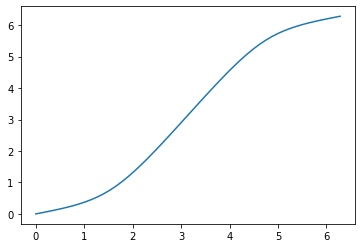The curve has exactly the shape we expect: the planet moves slowly at first, accelerates to its maximum speed (steepest slope) at 𝜏/2 — the point on its orbit farthest from the equant — then slows again until it reaches its starting point at an angle of 𝜏.

The tool that CSS offers for controlling non-linear animation is a cubic Bézier Curve starting at the coordinate (0,0) and ending at (1,1). Substituting these fixed coordinates into the generic formula on the Wikipedia’s Bézier Curve page, we get:

```def bezier(t, x1, y1, x2, y2):
m = 1 - t
p = 3 * m * t
b, c, d = p * m, p * t, t * t * t
return (b * x1 + c * x2 + d,
b * y1 + c * y2 + d)```

The curve’s formula is specially designed so that the curve leaves the origin with an angle and velocity determined by the line from (0,0) to (x₁,y₁), then dives in towards its destination at an angle and speed determined by the line from (x₂,y₂) to (1,1). For example, we can ask the curve to launch out at a low angle along the x-axis and then, at the top of the diagram, to finish with a similar horizontal approach to its endpoint — producing a spline that reminds us of the equant’s motion:

```t = linspace(0, 1)
xb, yb = bezier(t, 0.8, 0, 0.2, 1)
plot(xb, yb, '.-')```
`[<matplotlib.lines.Line2D at 0x7f617d9e7b38>]`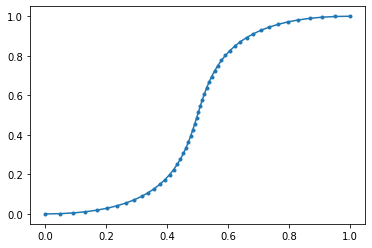Can Python help us refine the positions of the points (x₁,y₁) and (x₂,y₂) so the spline comes very close to the shape of the equant?

SciPy provides a `curve_fit()` function that searches for the parameters that will make one curve as close as possible to the shape of a target curve, but it carries a requirement: the target curve and the test curve both need to provide y values that correspond to the same set of x’s — or, in our case, to the same input M array.

The Bézier curve we’ve produced, alas, does not satisfy this criterion. A Bézier curve is rather independently minded about both its x’s and its y’s. Driven by its input parameter t, the Bézier’s output x and y coordinates make their own decisions about whether to space themselves close together or far apart as the curve swings from its origin to its destination. You can see that the example curve above starts at a high velocity with points spaced far apart, slows down in the middle as the curve moves steeply upward and points come close together, then finishes by accelerating again to its destination at the upper right. We need x’s spaced evenly instead.

Fortunately, NumPy has a solution! It offers an interpolation routine that takes a curve as input and uses it to interpolate y positions for a new input array x. What happens if we ask for the Bézier curve to be mapped atop our input array M?

`plot(M, interp(M, xb, yb), '.-')`
`[<matplotlib.lines.Line2D at 0x7f617d94af60>]`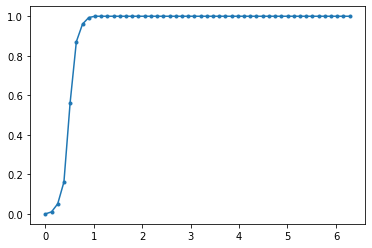Whoops.

The result looks rather comic: the entire Bézier curve has been squashed into only about one-sixth of the range of M. We forgot that our input arrays have two completely different domains: uniform circular motion M runs from 0 to 𝜏, while a CSS Bézier curve runs from 0 to 1.

The Bézier curve needs to be scaled up to cover the entire domain of M:

`plot(M, interp(M, xb * 𝜏, yb * 𝜏), '.-')`
`[<matplotlib.lines.Line2D at 0x7f617d8bd748>]`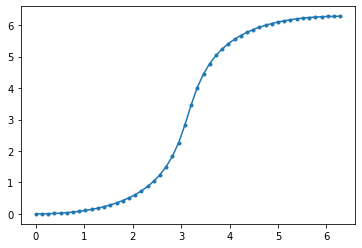To let `curve_fit()` do its job successfully, let's create a wrapper that scales points between M₀ and M₁ into the range [0,1] for input to the Bézier function, and whose output range [0,1] is then expanded to produce output between E₀ and E₁.

```def scaled_bezier(M0, M1, E0, E1):
def bez(M, x1, y1, x2, y2):
t = linspace(0, 1)
xb, yb = bezier(t, x1, y1, x2, y2)
x = (M - M0) / (M1 - M0)
y = interp(x, xb, yb)
return E0 + (E1 - E0) * y
return bez```

We can now ask SciPy to find a Bézier curve that approximates the equant:

```from scipy.optimize import curve_fit

bez = scaled_bezier(0, 𝜏, 0, 𝜏)
guess = [0.5, 0.0, 0.5, 1.0]
args, v = curve_fit(bez, M, E, guess, bounds=[0,1])
args```
`array([0.47532029, 0.06537691, 0.52467971, 0.93462309])`

Success! The Bézier curve, shown here by orange dots, looks like a fairly close approximation for the equant:

```plot(M, E)
plot(M, bez(M, *args), '.')```
`[<matplotlib.lines.Line2D at 0x7f617d97ed68>]`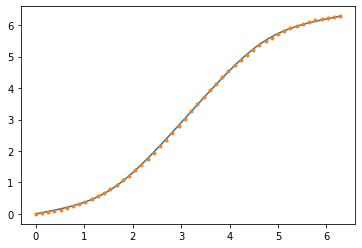How closely will the resulting animation represent the true rotation of the equant?

`max(abs(E - bez(M, *args))) * 360 / 𝜏`
`1.980801996224498`

This indicates that at the worst spots, this curve will lead or lag the true rotation by almost 2° of arc. Will that be noticeable? Let’s take a look! Here’s CSS that will use a custom animation function to control rotation speed:

```bezier1_css = '''
<style>
.bezier1 {
animation-name: bezier1;
}
@keyframes bezier1 {
from {animation-timing-function: %s}
to {transform: rotate(360deg)}
}
</style>
'''```

And a routine to properly format the function:

```def cb(args):
argstr = ', '.join('%.3f' % n for n in args)
return 'cubic-bezier(%s)' % argstr

cb(args)```
`'cubic-bezier(0.475, 0.065, 0.525, 0.935)'`

Does the result produce an equant and a planet that appear to rotate together?

```css = bezier1_css % cb(args)
HTML(equant_svg % ('bezier1', 70, 170) + css)```

Drat.

The result is uninspiring. The planet clearly lags behind, then moves ahead of, the target set by the sweeping line of the equant. It turns out that 2° is pretty noticeable.

There is an old rule for approximating motion with a Bézier curve: if one curve doesn’t work, then try more!

I tried two curves; the improvement was not enough. So I tried three, and the result is much better. Here’s a quick loop that splits our curve into three at the M values 𝜏/3 and 2𝜏/3, and finds the Bézier parameters for each third of the curve. The result will be 3 `keyframes` that run in succession, each controlled by a different Bézier curve.

```KEYFRAME = '''\
%.3f%% {
animation-timing-function: %s;
}
'''

boundaries = [0, 𝜏/3, 2*𝜏/3, 𝜏]
keyframes = []
for M0, M1 in zip(boundaries[:-1], boundaries[1:]):
M = linspace(M0, M1)
E = equant_E(M, 0.7)
bez = scaled_bezier(M0, M1, E, E[-1])
args, v = curve_fit(bez, M, E, bounds=[0,1])
percent = M0 / 𝜏 * 100
keyframe = KEYFRAME % (percent, E, cb(args))
keyframes.append(keyframe)

print(''.join(keyframes))```
```0.000% {
animation-timing-function: cubic-bezier(0.480, 0.206, 0.681, 0.333);
}
33.333% {
animation-timing-function: cubic-bezier(0.298, 0.264, 0.702, 0.736);
}
66.667% {
animation-timing-function: cubic-bezier(0.319, 0.667, 0.520, 0.794);
}

```

The CSS otherwise looks the same as before.

```bezier2_css = '''
<style>
.bezier2 {
animation-name: bezier2;
}
@keyframes bezier2 {
%s
to {transform: rotate(360deg)}
}
</style>
'''```

But, this time, the visual output is stunning! The planet seems to follow the equant’s motion perfectly, moving slowly when close to the equant point, and very rapidly when on the opposite side of its orbit.

```css = bezier2_css % ''.join(keyframes)
html = equant_svg % ('bezier2', 70, 170) + css
HTML(html)```

And the best part is that the animation does not involve matplotlib, isn’t delivered as raster graphics, and won’t involve a trade-off between file size and visual quality. Instead, we’ve delivered it to the browser using less than 1k characters!

`len(html)`
`892`

And in return we enjoy a crisp and accurate animation.

With this technique, I'm now ready to move forward! Finally, after these years of delay, I’ll use my next few blog posts to build from these simpler pieces a complete Ptolemaic model of the solar system — all animated in the browser without any matplotlib involved.